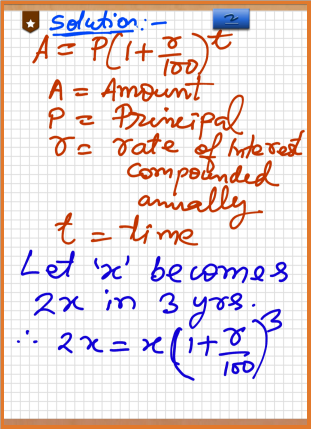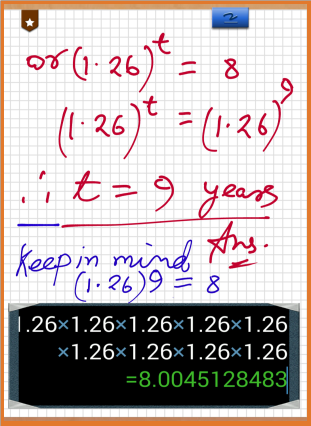Mar 4, 2015

# A sum of Money becomes double in 3 years | At a compound rate of interest | What is rate of Interest | In how many times it will become 8 times

Sometimes this type of sum are given by the teaches in which logarithm cannot be used at grade or class 8 level. Teacher want to puzzle students so that more students join to their private coaching. You need not to worry . We are here to help the needy students. Let first reed the Sum .

This sum will be shown by two method. first one is preferable for students of class 8 to class 10

Question:- A sum of money becomes double in 3 years at a compound rate of interest. Howmany years It will be its 8 times at the same rate. Find the compound rate of interest and the time in which it becomes its 8 times.

1st method

solution: Let 'x' becomes 2x in 3 years. We know that : A=P(1+r/100)t A=Amount
P= Principal
r=compound rate of interest
t=time
∵ 2x=x (1+r/100)3
∴ 2 = (1+ r/100)3
Now 8x=x(1+r/100)t
8 = (1+r/100)t
Or 2 3= (1+ r/100)t
or [(1+ r/100)3]3=(1+r/100)t
or (1+ r/100)3x3=(1+r/100)t
(1+ r/100)9=(1+r/100)t
Now the base (1+ r/100) is same at both side.
Therefore, t = 9 years. ANS.

2nd method

∵ 2x=x (1+r/100)3
∴ 1+ r/100=(2)(1/3)
∴ 1+r/100=1.2599
∴ r=26
Now 8x=x(1+26/100)^t
or 8=(1.26)^t
or (1.26)^9=(1.26)^t
Therefore, t=9 years. ANS.# You may also Like These !